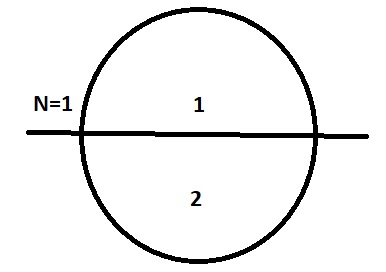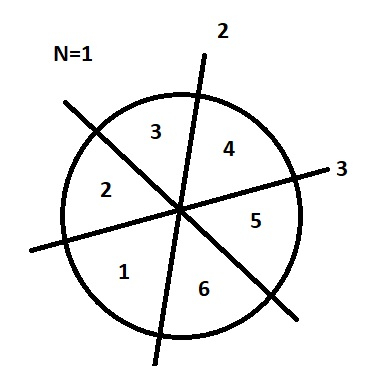# Count pieces of the circle after N cuts in C++

We are given an integer N which represents the number of cuts applied on a 2D-circle. Each circle divides the circle in two halves. Goal is to find the pieces of the circle after N cuts.

Number of pieces= 2 * no. of cuts

Let’s understand with examples.

Input − N=1

Output − Pieces of circle: 2

ExplanationInput − N=3

Output − Pieces of circle: 6

Explanation## Approach used in the below program is as follows

• We take N for a number of cuts.

• Take pieces=1*N.

• Print the result..

## Example

#include <bits/stdc++.h>
using namespace std;
int main(){
int N=2;
Int pieces=2*N;
cout <<endl<<”Number of pieces of circle: ”<<pieces;
return 0;
}

## Output

If we run the above code it will generate the following output −

Number of pieces of circle: 4